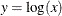### BOUNDS Statement

BOUNDS constraint <, constraint …> ;

where constraint represents < number operator > parameter-list < operator number >

You can use the BOUNDS statement to define boundary constraints for any independent parameter that has its name specified in the main or subsidiary model specification statements, the PARAMETERS statement, or the INMODEL= data set. You cannot define boundary constraints for dependent parameters created in SAS programming statements or elsewhere.

Valid operators are <=, <, >=, >, and = (or, equivalently, LE, LT, GE, GT, and EQ). The following is an example of the BOUNDS statement:

bounds        0.   <= a1-a9 x    <= 1. ,
-1.   <= c2-c5            ,
b1-b10 y   >= 0. ;


You must separate boundary constraints with a comma, and you can specify more than one BOUNDS statement. The feasible region for a parameter is the intersection of all boundary constraints specified for that parameter; if a parameter has a maximum lower boundary constraint greater than its minimum upper bound, the parameter is set equal to the minimum of the upper bounds.

The active set strategies made available in PROC CALIS treat strict inequality constraints < or > as if they were just inequality constraints <= or >=. For example, if you require x be strictly greater than zero so as to prevent an undefined value for, specifying the following statement is insufficient:

BOUNDS x > 0;

Specify the following statement instead:

BOUNDS x > 1E-8;

If the CALIS procedure encounters negative variance estimates during the minimization process, serious convergence problems can occur. You can use the BOUNDS statement to constrain these parameters to nonnegative values. Although allowing negative values for variances might lead to a better model fit with smallervalue, it adds difficulties in interpretation.